#### You may also like### Multiplication Magic

Given any 3 digit number you can use the given digits and name another number which is divisible by 37 (e.g. given 628 you say 628371 is divisible by 37 because you know that 6+3 = 2+7 = 8+1 = 9). The question asks you to explain the trick.### Two Cubes

Two cubes, each with integral side lengths, have a combined volume equal to the total of the lengths of their edges. How big are the cubes? [If you find a result by 'trial and error' you'll need to prove you have found all possible solutions.]### Common Divisor

Find the largest integer which divides every member of the following sequence: 1^5-1, 2^5-2, 3^5-3, ... n^5-n.

##### Age 14 to 16 Challenge Level:

Luke from London Oratory School explained how the situation can be shown using algebra: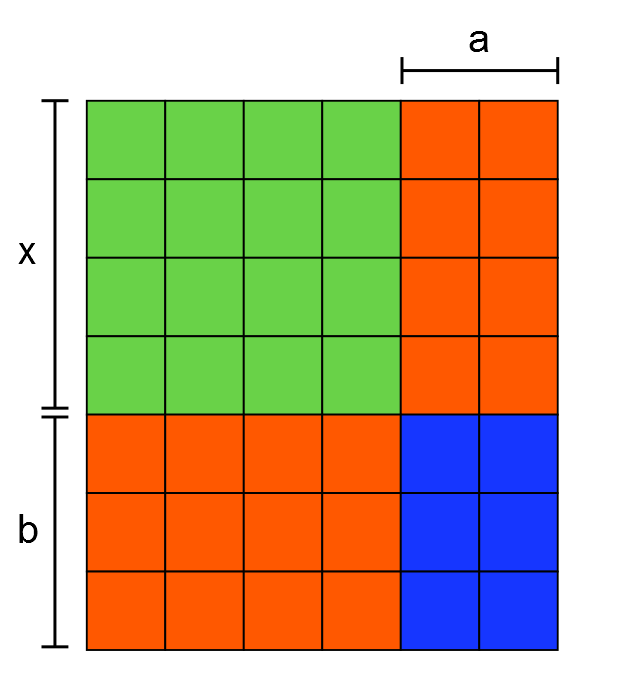The green section is a square, so its area is equal to $x^2$.
The red section (made of sticks) consists of two rectangles with dimensions $a, x$ and $b, x$. Therefore the red area is equal to $ax+bx$, which equals $x(a+b)$.
We can see that the area of the blue section will always have dimensions $a$ and $b$, so its area is equal to $ab$, if it ʻﬁllsʼ the gap created by the red area.
The total area is equal to the sum of these component areas.
Thus you can make a rectangle for all bases for expression of the form $x^2 + x(a+b) + ab$ where $a$ and $b$ are positive integers. The rectangle has dimensions $(x+a)$ by $(x+b)$.

1 square, lots of sticks and 12 or 100 units
Mahdi, Hamaad and Khalid used algebra again. Here is Mahdi's work for 12 sticks: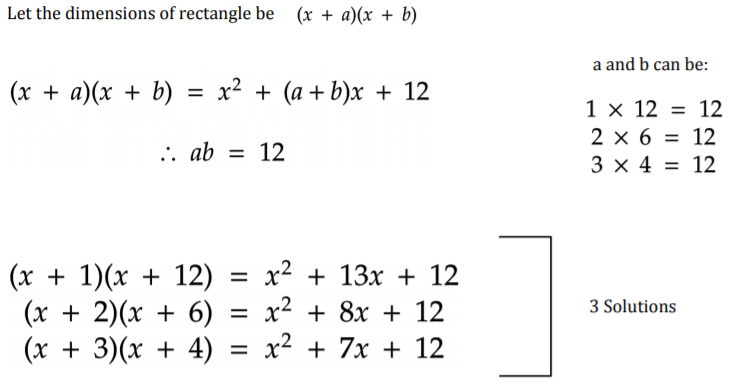This is Hamaad and Khalid's work for 100 sticks: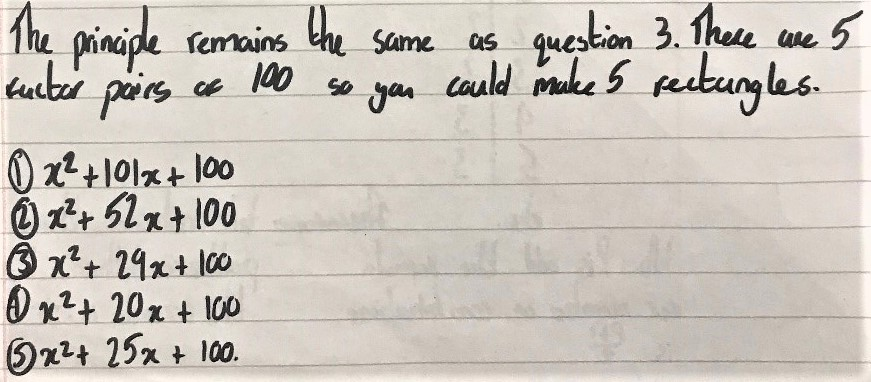1 square, 12 or 100 sticks and lots of units
Hadi from Michaela Community School in the UK started with smaller numbers of sticks and then spotted a pattern: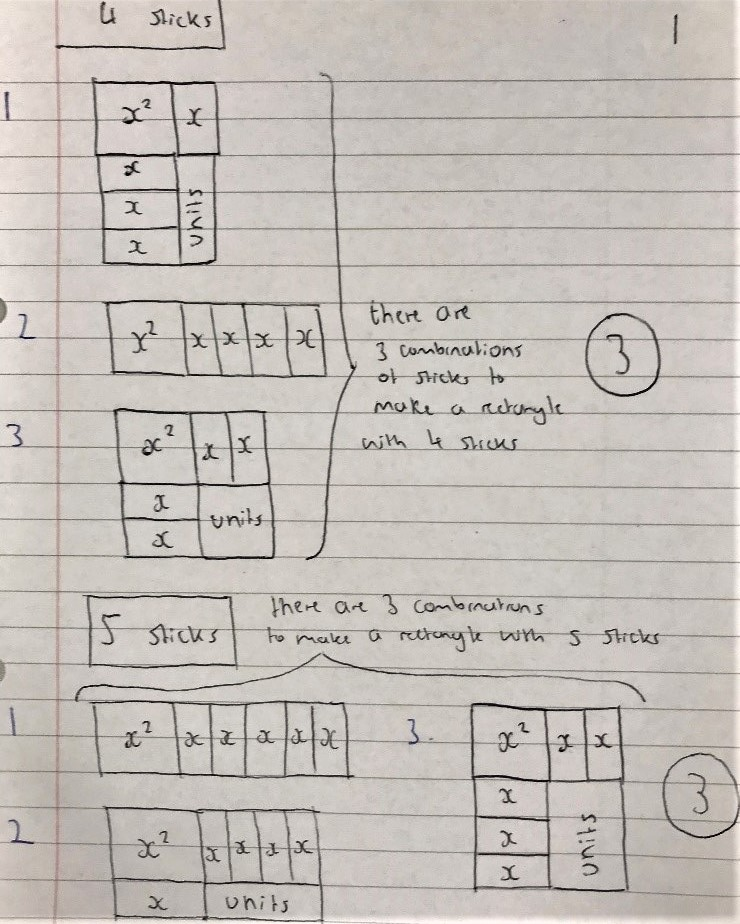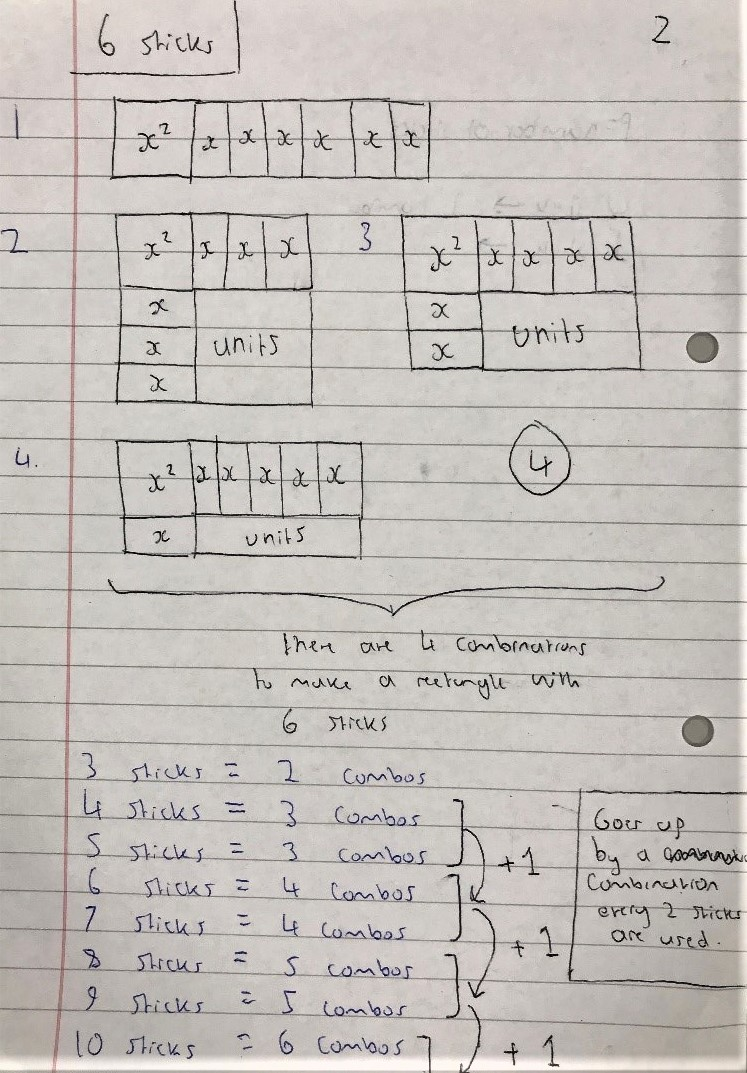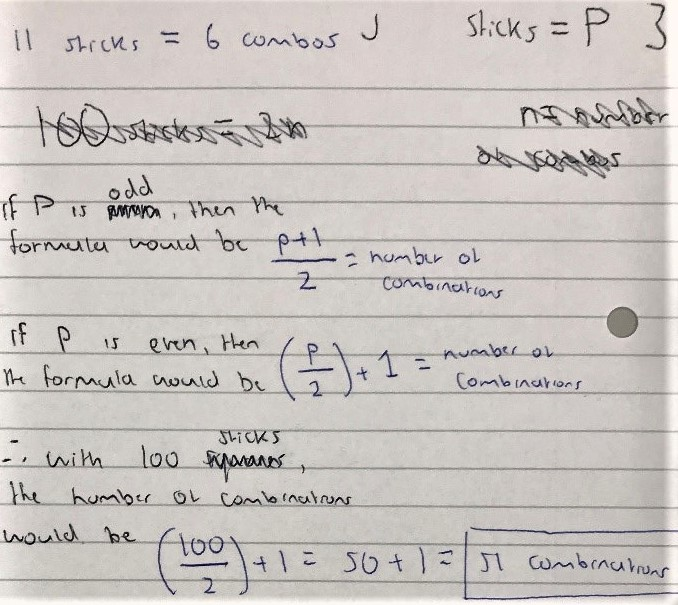Mahdi from Mahatma Gandhi International School in India used algebra: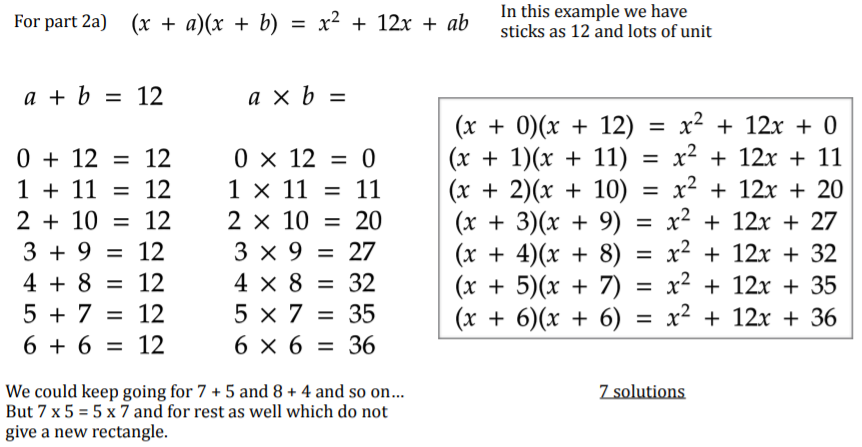Hamaad and Khalid from Michaela Community School and Mahdi used the same approah for 100 sticks. This is Hamaad and Khalid's work: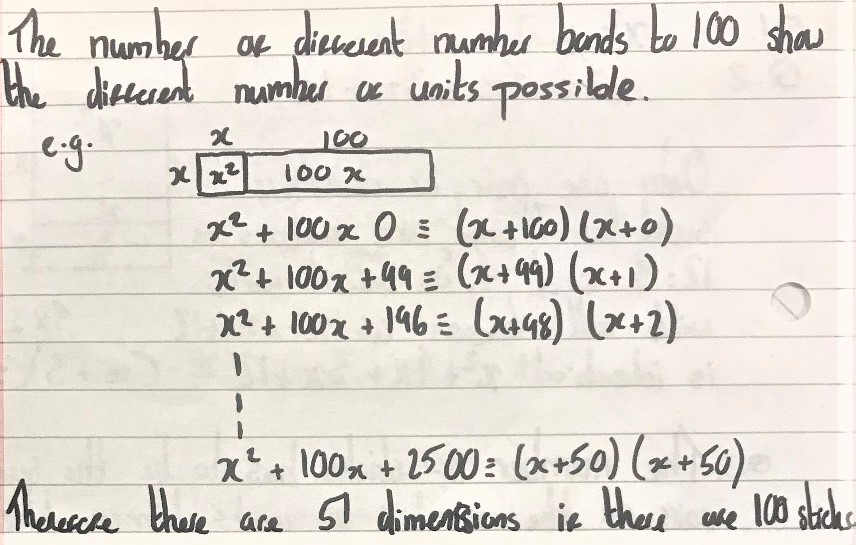For $p$ sticks and $q$ units
Mahdi approached this algebraically: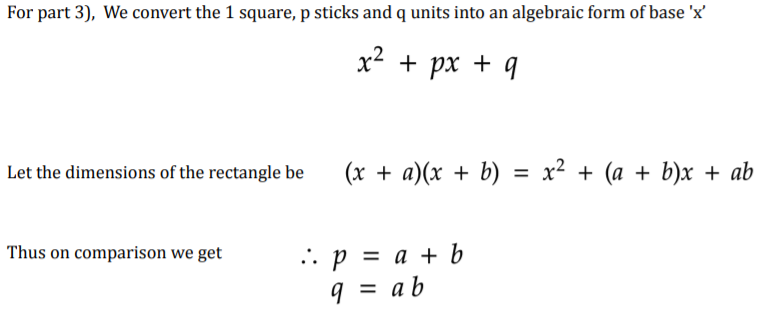So how many different rectangles can you make with $p$ sticks and $q$ units?